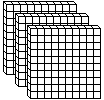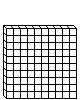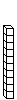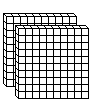Create a new printable
Answer key also includes questions
Answer key only gives the answers
No answer keyMath Worksheets

Sample - Click above to make a new math worksheet (PDF).
 Name _____________________________Date ___________________
Hundreds and Tens
Write the number of hundreds and tens. Write the number.

1.___ hundreds  ___ tens  ___ ones Write the number: ______
2.___ hundreds  ___ tens  ___ ones Write the number: ______
 3 * This is a pre-made sheet.Use the link at the top of the page for a printable page.
4.___ hundreds  ___ tens  ___ ones Write the number: ______
5.___ hundreds  ___ tens  ___ ones Write the number: ______

 Answer Key

Sample
This is only a sample worksheet.

Create a new printable
Answer key also includes questions
Answer key only gives the answers
No answer keyMath Worksheets